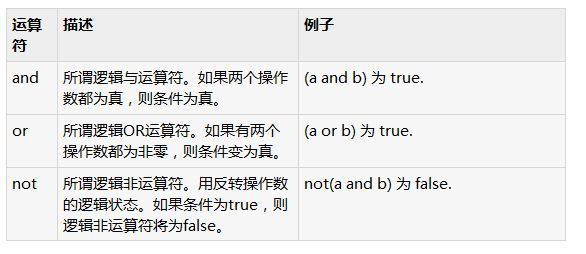# 总结Python中逻辑运算符的使用```#!/usr/bin/python

a = 10
b = 20
c = 0

if ( a and b ):
print "Line 1 - a and b are true"
else:
print "Line 1 - Either a is not true or b is not true"

if ( a or b ):
print "Line 2 - Either a is true or b is true or both are true"
else:
print "Line 2 - Neither a is true nor b is true"

a = 0
if ( a and b ):
print "Line 3 - a and b are true"
else:
print "Line 3 - Either a is not true or b is not true"

if ( a or b ):
print "Line 4 - Either a is true or b is true or both are true"
else:
print "Line 4 - Neither a is true nor b is true"

if not( a and b ):
print "Line 5 - Either a is not true or b is not true"
else:
print "Line 5 - a and b are true"

```

```Line 1 - a and b are true
Line 2 - Either a is true or b is true or both are true
Line 3 - Either a is not true or b is not true
Line 4 - Either a is true or b is true or both are true
Line 5 - Either a is not true or b is not true

```

Tools Online | 在线开发工具
RankList | 热门文章Recommend | 推荐阅读
PHP1.CN | 中国最专业的PHP中文社区 | PHP资讯 | PHP教程 | 数据库技术 | 服务器技术 | 前端开发技术 | PHP框架 | 开发工具 | PHP问答